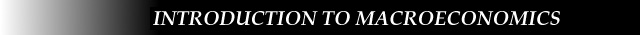## Chapter Ten: Module Intro -- The Fixed Price AD/AS Model

We examined the Aggregate Demand/Aggregate Supply model in Chapter 8, and the Income-Expenditure model in Chapter 9. In this chapter, we bridge the gap explicitly between the two models by analyzing one special case of the Aggregate Demand/Aggregate Supply model -- the case in which the Aggregate Supply curve is perfectly horizontal. This scenario is appropriate when studying the very short run and when the economy is in a recessionary gap. When the Aggregate Supply curve is horizontal, shifts in the Aggregate Demand curve lead only to changes in output; the price level is unaffected.

Read the lecture notes first, then take the on-line quiz. Finally, review the module summary to reinforce the concepts.